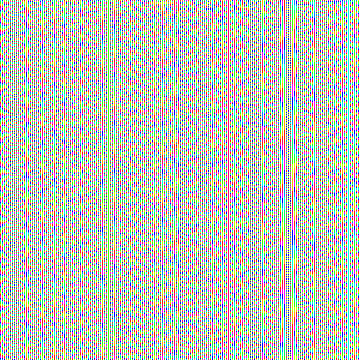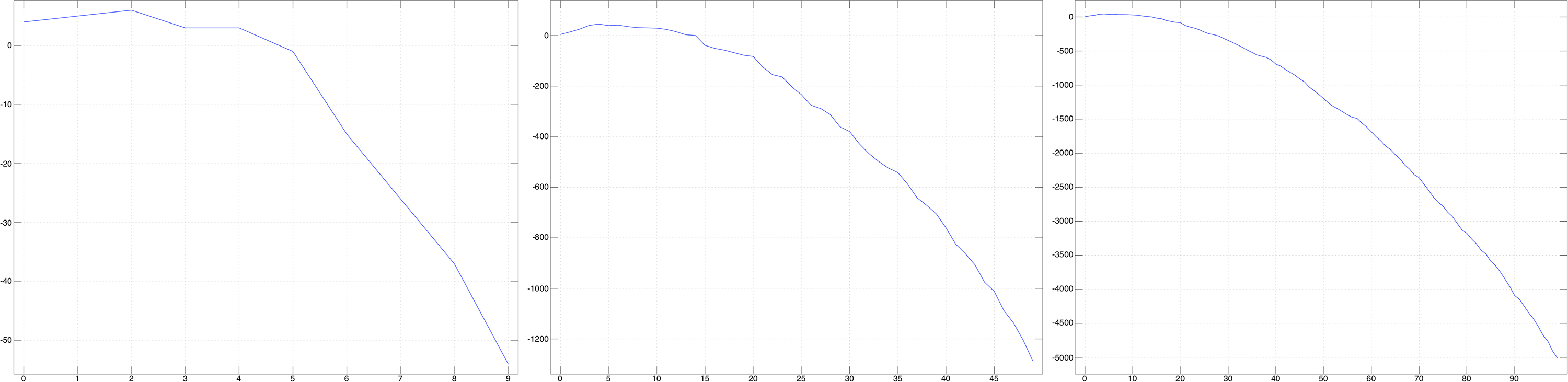Advent of Code’s day eleven puzzle asks us to compute a three hundred square grid of values and to find the three by three sub-grid that contains the highest sum of values. In my heart of hearts I knew that this would be a problem well suited to the J programming language.

I started by working on a verb to break a grid into a number of sub-grids of a given size. This reminded me quite a bit of Elixir’s `Enum.chunk_every/4` function, so I decided to name it accordingly:

``````    grid =. 5 5 \$ i. 25
grid
0  1  2  3  4
5  6  7  8  9
10 11 12 13 14
15 16 17 18 19
20 21 22 23 24
chunk_every =. [ <\"2 [: |:"2 [: > <\
3 chunk_every grid
┌────────┬────────┬────────┐
│0 5 10  │1 6 11  │2 7 12  │
│1 6 11  │2 7 12  │3 8 13  │
│2 7 12  │3 8 13  │4 9 14  │
├────────┼────────┼────────┤
│5 10 15 │6 11 16 │7 12 17 │
│6 11 16 │7 12 17 │8 13 18 │
│7 12 17 │8 13 18 │9 14 19 │
├────────┼────────┼────────┤
│10 15 20│11 16 21│12 17 22│
│11 16 21│12 17 22│13 18 23│
│12 17 22│13 18 23│14 19 24│
└────────┴────────┴────────┘
``````

To be totally transparent, I originally came up with this verb by tinkering in the REPL, and converted it into a tacit verb after the fact.

Now that we have `chunk_every`, we can define a few more needed values, like our initial grid, our grid size, and our grid serial number:

``````    size =. 300
grid =. (size,size) \$ i. size * size
grid_serial_number =. 9424
``````

The puzzle tells us how to convert our initial grid’s x/y coordinates into “fuel cell values”. Let’s write an `init` verb that takes our initial verb and calculates and populates those values:

``````    init =. 3 : 0
'cy cx' =. (0,size)#:y
rack_id =. cx + 10
power =. rack_id*cy
power =. power + grid_serial_number
power =. power * rack_id
power =. 10 | power <.@% 100
power =. power - 5
power
)
``````

Now we’re ready to start. We’ll begin by generating our grid of fuel cells:

``````    grid =. init"0 grid
``````

Next, we’ll break our grid into three by three chunks:

``````    chunks =. 3 chunk_by grid
``````

Once we have our sub-grids, we’ll calculate the sum of each and flatten that into a one dimensional array of sums:

``````    flat_chunks =. , +/"1 ,"2 > chunks
``````

And find the maximum sub-grid sum:

``````    max =. >./ flat_chunks
``````

And the corresponding index of that maximum sum:

``````    highest =. flat_chunks i. >./ flat_chunks
``````

Finally, we can turn that index into a pair of x/y coordinates:

``````    |. (0,(size-2))#:highest
``````

This is the answer to our puzzle. Victory!Our fuel cells, visualized.

Just for fun, we can check out what our newly initialized fuel cell matrix looks like with the help of `viewmat`. We can see some cool Moiré patterns in the resulting visualization as a side effect of our calculations.

## Part Two

Part two wants us to vary the size of our sub-grids, and find the sub-grid size, and x/y coordinate pair that has the most amount of available fuel, or the highest sum.

My first instinct was to run `chunk_by` multiple times against chunk sizes ranging from `1` to `300`:

``````    chunks =. (grid&(<@:chunk_by~))"0 (1 + i. size)
``````

I wrote a verb to `count` the amount of available fuel within each new set of sub-grids, and ran that against all of the sub-grid sets I was generating:

``````    count =. 3 : 0
chunks =. > y
chunk_size =. # 0 0 {:: chunks
flat_chunks =. , +/"1 ,"2 > chunks
max =. >./ flat_chunks
highest =. flat_chunks i. >./ flat_chunks
coord =. |. (0,(size-(chunk_size-1)))#:highest
max;coord,chunk_size
)

counts =. count"_1 chunks
``````

From there, I could grab the best score of all of the sub-grid sizes, find the max, and return a tuple of that winning sub-grid’s size, and its x/y coordinate:

``````    scores =. > 0 {"1 counts
idx =. scores i. >./ scores
1 {:: idx { counts
``````

Unfortunately, this turned out to be too slow of a solution.

Thankfully, there were some patterns to be found that let us speed things up considerably! If we `plot` our `counts` with a max grid size of `10`, `50`, and `100`, we can see that our score always peaks almost immediately. We don’t need to test larger sub-grid sizes because we know our answer won’t be there.A plot of our sub-grid size vs our maximum fuel availability.

Let’s change our solution to only check sub-grid sizes from one to twenty:

``````    chunks =. (grid&(<@:chunk_by~))"0 (1 + i. size)
``````

And just like that, we get an answer almost immediately.

Thanks J!